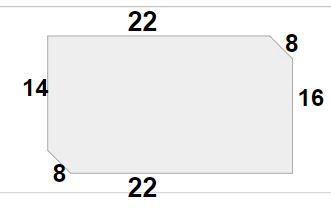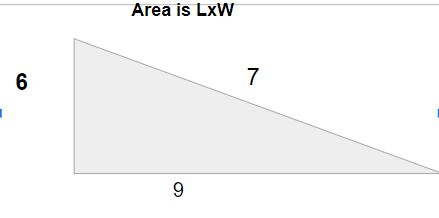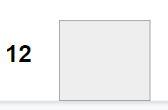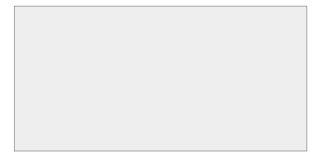Perimeter
Area
Operations
Random Math
100

What is Perimeter?

Perimeter is the measure of the outside lengths of a shape

100

What is area?

Area is the measure of the inside of a shape

100

What operation do i use to find the sum?

100

45/5=?

9

200

How do i solve perimeter?

200

How do a find area?

by multiplying length times width

lxw=a

200

If Sally has 18 apples but 4 have be thrown away, how many does she have left?

What happens if she is given 15 apples later?

18-4=14

14+15=29

200

17+26

33

300

Find the perimeter. of the shapebelow

14+8+8+16+22=68

300

Find the area of the triangleL=6

W=9

6X9=654

300

What operations tell me to make something?

300

20x10

200

400

How many equal sides does this polygon have ?4 equal pairs of sides

400

Find the area of the squareL=12

W=12

12x12=144

400

Multiplication is the inverse of _______

Inverse=opposite

The inverse of addition is subtraction

The inverse of multiplication is division

400

Solve 15x6 using circles

15 circles with 6 dots in each, 90

500

Find the perimeter74

500l=17

w=17

lxw

17x17

500

Cassie has 14 pair of shoes. She receives 3 more pairs for her birthday.  How many pairs does Cassie have in total?

How many shoes in total?

Cassie has 17 pairs of shoes and

17x2=34 shoes all total

500

Solve the fact family

15/3=?

?x3=15

?=5

Click to zoom